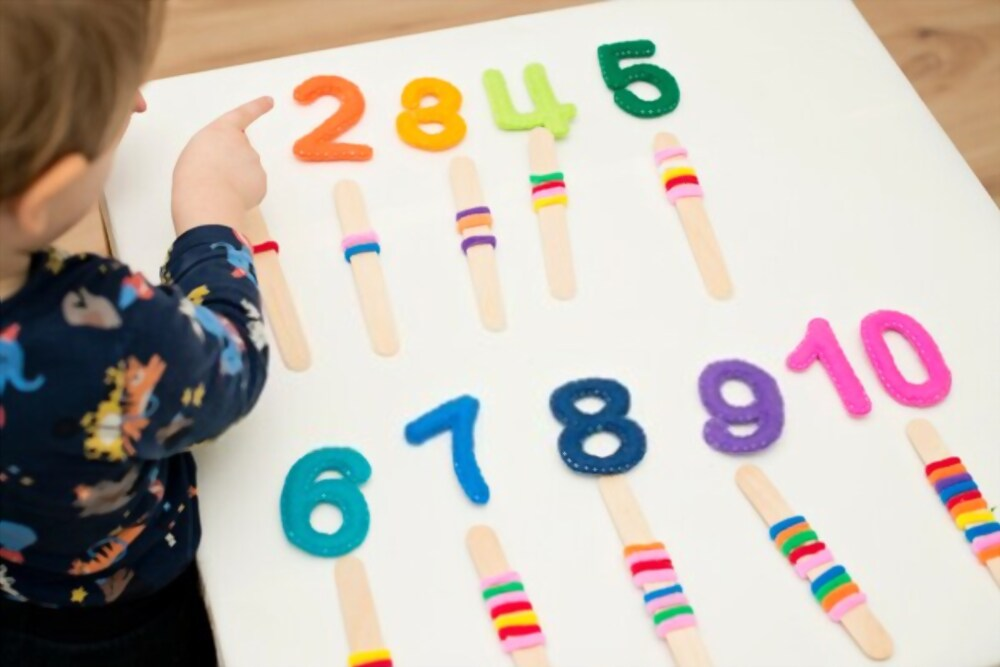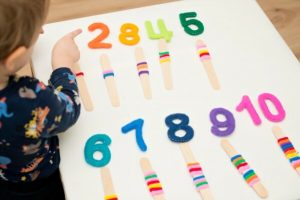# Number Series for Competitive Exams## What is a Number Series?

A series of numbers arranged in a certain way is called Number Series. Every Number of a series is arranged in some pattern or in some specific way, before answering the question make sure you understand it well. It is recommended to check whether all terms are satisfying with it or not.

Questions from Number series can be asked in following Manner—

1. Which will be the next term.
2. Find the missing term.
3. Find the wrong term.
4. Identify pattern of series I and complete series II.
5. Find the wrong series among 3 given series.
6. Few series and their missing terms are given, find the relation between these missing terms.

### 1. Which will be the next term

This type of Number series question is generally asked more in Exams. Under this type of question you have observe the given series carefully and then will answer the next term.

Most of the time these types of questions are based on Difference  or multiple, Square, Cubes, AP, GP and other such operations. Series of Prime Numbers, Odd Numbers, Even Numbers, Natural Numbers, Integers etc. can also be given to complete

For Example: 2, 4, 6, 8, ?

If we observe the above mentioned series carefully then we will find that here a series or first few Even number is given (or you can also say that there is a gap or difference of 2 in each Term)

so the next Term of the series must be 10.

### 2. Find the missing term.

In this type of Series we have to find the Missing term of the series. For predicting or answering the missing term of the series correctly, we have to observe it carefully and will have to understand the pattern first.

In this type of questions too, most of the time questions  based on Difference  or multiple, Square, Cubes, AP, GP and other such operations like Series of Prime Numbers, Odd Numbers, Even Numbers, Natural Numbers, Integers etc. are given.

For example: 5, 10, 20, _, 55, 80.

If we observe the above mentioned Series carefully then we will find that there is a gap of Multiple of 5, between each terms of serious.

So the missing term of the series must be 35

### 3.Find the wrong term

This type of the Number series question is mostly hated by the candidates. Because you have to understand the correct pattern and while observing the pattern one can considered the wrong term as correct and because of this it takes more time.

For example: 1, 8, 27, 66, 125, 216, 343.

If we observe the above mentioned series carefully then we will find that Cubes of first 7 Natural Numbers. Observing carefully we will find that Cube of 4 is 64 not 66.

So the Wrong term of the series is 66.

### 4. Identify pattern of series I and complete series II

These type of Series questions are mostly asked in Mains Exams. First Series based on certain pattern is given and first term of Series 2 is given and the candidate has to either find rest terms of series 2 or any one term of series 2.

For Example: Series I – 2, 4, 8, 16, 32, 64, 128.

Series II – 5, 10, _, _, _, _.

If we observe the above mentioned Series Carefully then we will observe that each term of series I is twice the previous one.

So the Terms of Series II – 20, 40, 80, 160, 320.

### 5. Find the wrong series among 3 given series.

These kind of questions are generally asked in Mains exams. Under these type of question 3 series of different pattern based will be given to you among them one series will have few wrong term because of which that will become a wrong series. Candidates were asked to identify that wrong series.

For Example:- Series I  10, 20, 30, 40, 50, 60.

Series II  1000, 1500, 500, 2000, 0, 3500.

Series III 1000, 729, 324, 343, 216, 125.

If we observe the following series carefully then we will observe that First series has difference of 10 among each term of the series. Whereas Sum and then Difference of multiple of 500 is there between each consecutive term of the series except 0 & 3500 where gap of 2500 was correct, and the last series is the cube of 10 to 5 consecutive Natural number.

So clearly here series 2 with wrong term of 3500 is a wrong series.

### 6. Few series and their missing terms are given, find the relation between these missing terms.

These kind of series is now asked in Mains Exams. although it takes time to solve but it is really a very scoring topic. Under this kind of question few series with few missing term will be given to you. After finding out the missing terms you will asked to write about the relation between them.

For Example: Series I – 3, 6, 9, 12, a, 18.

Series II – 100, 50, 25, b, 6.25, 3.125

Series III – 5, 25, c, 625, 1225, 3125.

If we observe  the above mentioned Series carefully then we will noticed that First series is based on multiple of 3. In second series each next term is a half of its preceding one, and Third series is based on 5^n , where n is natural number.

So the Value of a = 15, b= 12.5, & c=125.

so the relation between a, b, & c = c>b>a.